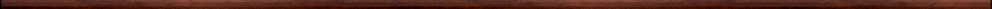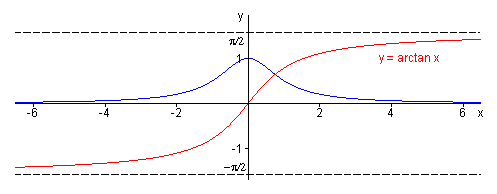Maple worksheets on integration techniquesBasic calculus topics:

They are all compatible with Classic Worksheet Maple 10.Changing variables in integrals - changevar.mws

• Using the Maple procedure changevar.
• Miscellaneous substitutions.
• Integrals of even and odd powers of sine and cosine.

Inverse Trigonometric Functions - invtrig.mws

• The arcsine function.
• The arccosine function.
• The arctangent function.
• A definite integral involving arctan.
• A definite integral involving arcsin.
• Standard integrals involving inverse trig functions.
• The area under a semicircle.

Integration of rational functions using partial fractions .. I - parfrac1.mws

• Area under the graph y=1/x.
• Introduction to the integration of rational functions.
• Examples of integrals involving partial fraction expansions with distinct linear factors in the denominator.
• The integral of the rational form f '(x)/f(x).
• More examples of integrals of rational functions.
• The integral with respect to x of 1/(a x + b) is ln(|a x + b|)/a +c .. examples
• Partial fractions expansions for the case of repeated linear factors in the denominator.
• Partial fractions expansions for the case of repeated quadratic factors in the denominator.
• Degree of numerator greater than or equal to degree of the denominator.

Integration of rational functions using partial fractions .. II - parfrac2.mws

• More integration examples involving partial fractions.

Integration of rational functions using partial fractions .. III - parfrac3.mws

• Partial fractions expansions for the case of repeated linear factors in the denominator.
• The integral with respect to x of (p x + q)/(a x^2 + b x + c).
• Partial fraction expansions which involve irreducible factors in the denominator.
• Degree of numerator greater than or equal to degree of the denominator.

Integration of rational functions using partial fractions .. IV - parfrac4.mws

• The integral with respect to x of 1/(x^2+a^2)^n.
• Partial fractions expansions which involve repeated irreducible quadratic factors in the denominator.

Integration of rational functions using partial fractions .. V - parfrac5.mws

• The integral with respect to x of 1/(x^3+1).
• The integral with respect to x of 1/(x^4+1).
• The integral with respect to x of 1/(x^5+1).

Integration by parts - intparts.mws

• The integration by parts formula.
• A procedure for performing integration by parts: intbyparts.
• Examples.

Top of page

Main index